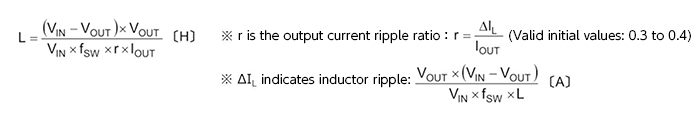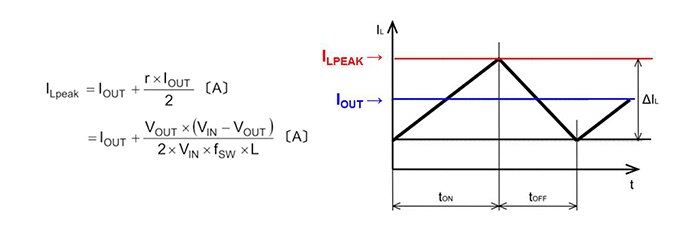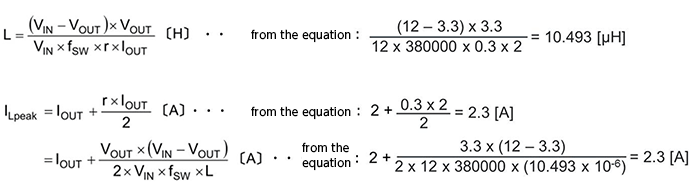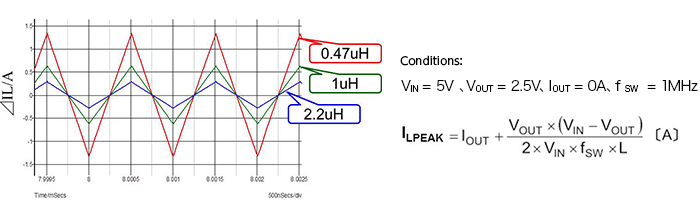Technical Information Site of Power Supply Design

2017.04.27 DC/DC

# Inductor Selection

Selection of Inductors and Capacitors for DC/DC Converters

When designing a step-down DC/DC converter, inductor selection is important. Inductor selection greatly influences performance and characteristics. The procedure for inductor selection and the method for calculating inductances and the like are basically indicated on the data sheets of the power supply IC that is used.

Inductor selection procedure

First, the procedure to use when selecting an inductor is described.

1）Calculate the required inductance L.

2）Calculate the maximum current flowing in the inductor (output current + one-half of the ripple
current).

3）Select an inductor having the calculated value L (or a value close to it) and with an inductor
saturation current at least as great as the calculated maximum current.
※In the event of a short-circuit or transient state, there are cases in which a current greater than
the calculated maximum current may flow; hence another method is to select the inductor based
on the maximum switch current.

In essence, the margin is considered and determined based on calculations. The method for determining the margin depends on the design rules of the company and empirical rules.

1）Inductance calculation
First, the following equations are used to calculate the inductance.2）Calculation of the maximum current of the inductor
Next, the maximum current of the inductor is calculated according to the following equation.As is clear from the equations and the current waveform, ILPEAK is equal to one-half of ΔIL with IOUTIOUT added.

From the calculated inductance and maximum current of the inductor, an inductor is selected having approximately the calculated inductance and a saturation current at least as large as the maximum current. Below an example of selection is described.

Example of Inductor Selection

Conditions: VIN = 12V, VOUT = 3.3V, IOUTIOUT = 2A, r = 0.3, f SW = 380kHzFrom the above results, the starting point will be an inductor of 10 μH, with a saturation current of at least 2.3 A. "Starting point" means that these calculations are not absolute, and modifications may be necessary when such things as short-circuits and transient states are taken into consideration.

Inductor Current when the Inductance is Changed

Here, in order to deepen our understanding of inductor operation, we explain the changes in the inductor current when the inductance is changed. The following chart shows ILPEAK when the inductance is set to 0.4 μH, 1 μH, and 2.2 μH, under the same operating conditions.As is also clear from the equation, when the inductance L is smaller, ILPEAK increases, and a larger superimposed direct current can be accommodated. However, the increase in ILPEAK necessitates tolerance of a larger superimposed direct current. If the inductance is made larger, the reverse applies, and there is the need to study phase compensation.

#### Key Points:

・Inductor selection is extremely important in DC/DC converter design.

・The circuit operation, current paths, and the relation between the inductor and the output current must be understood.

This website uses cookies.

By continuing to browse this website without changing your web-browser cookie settings, you are agreeing to our use of cookies.